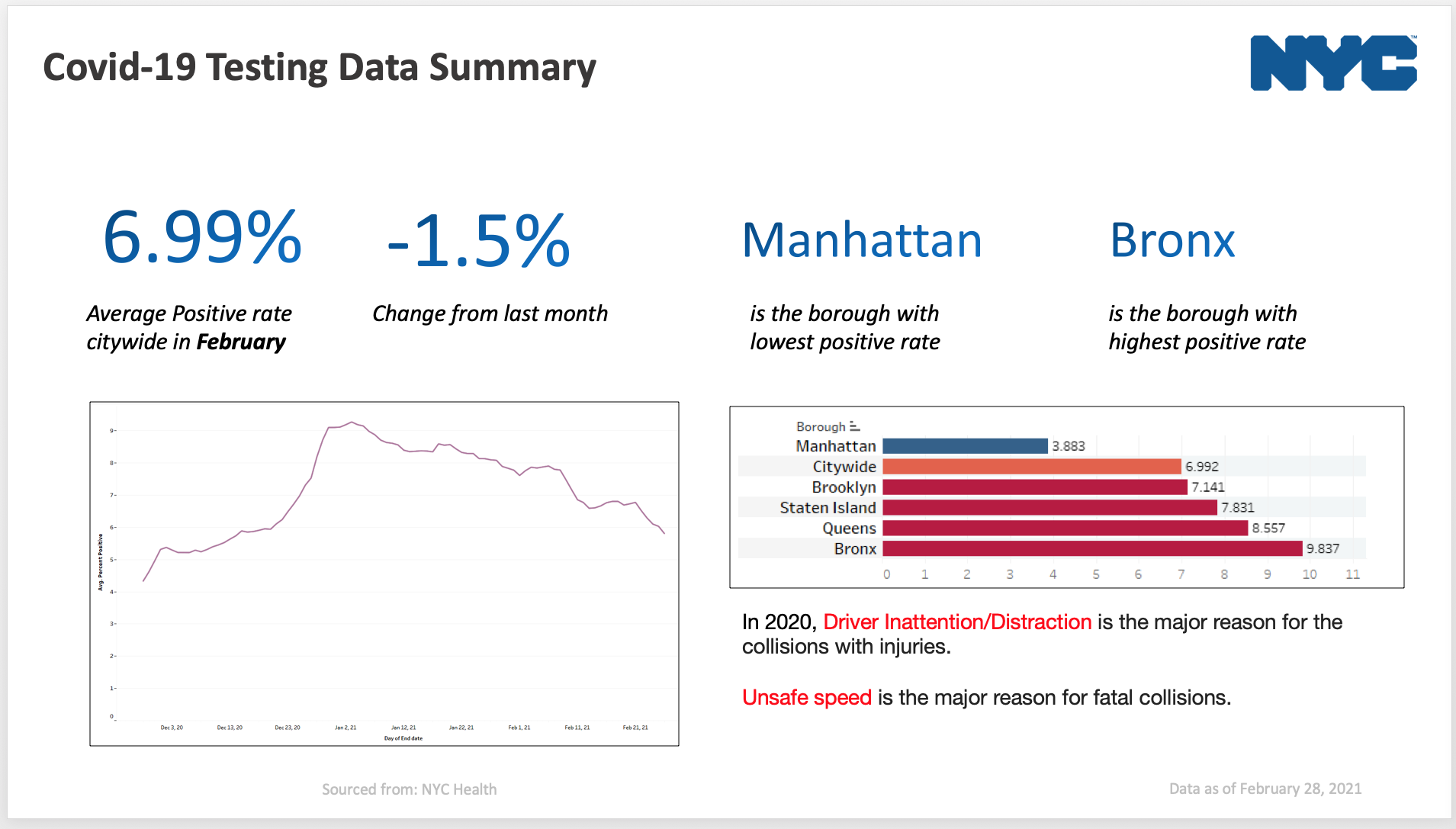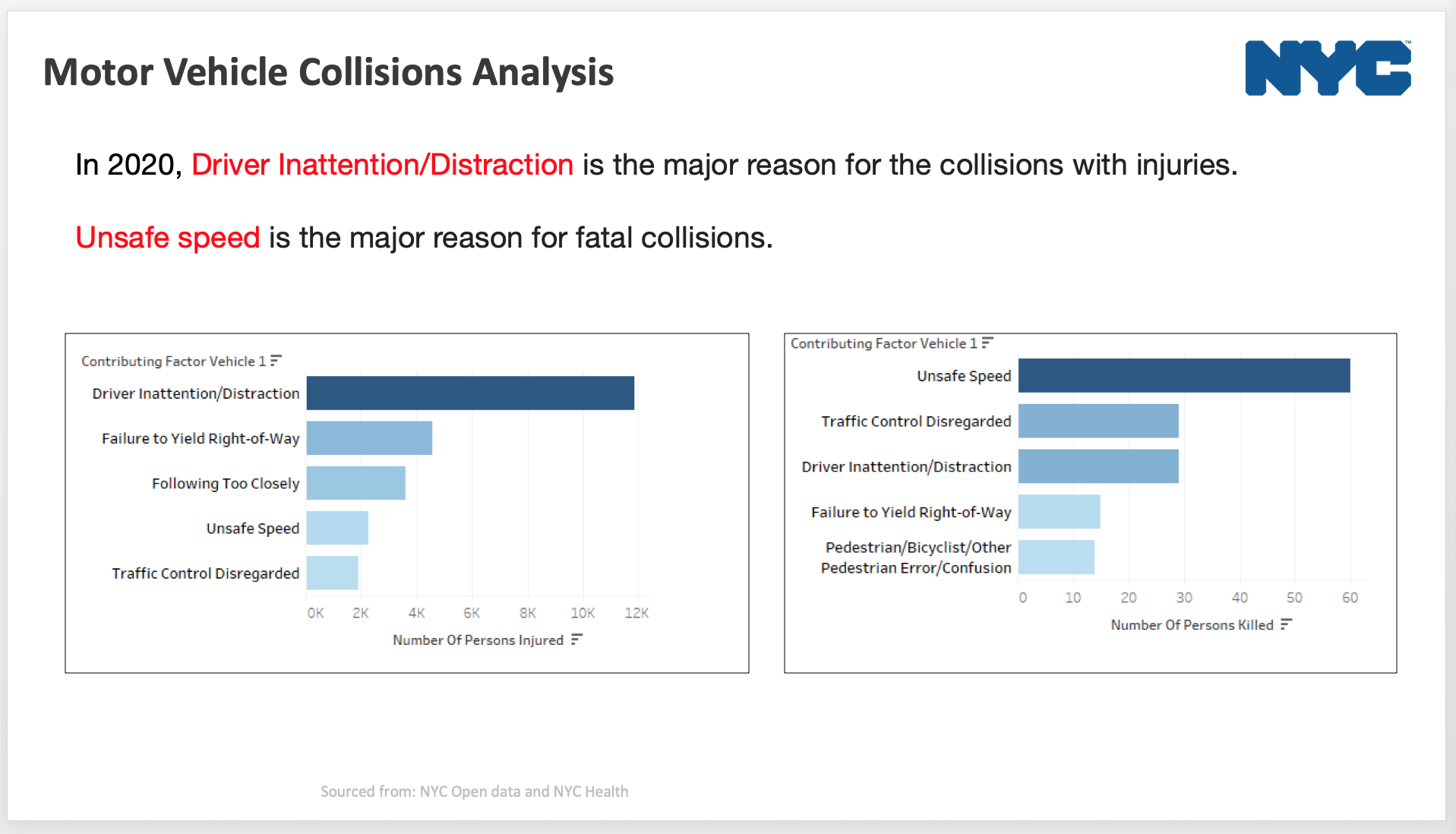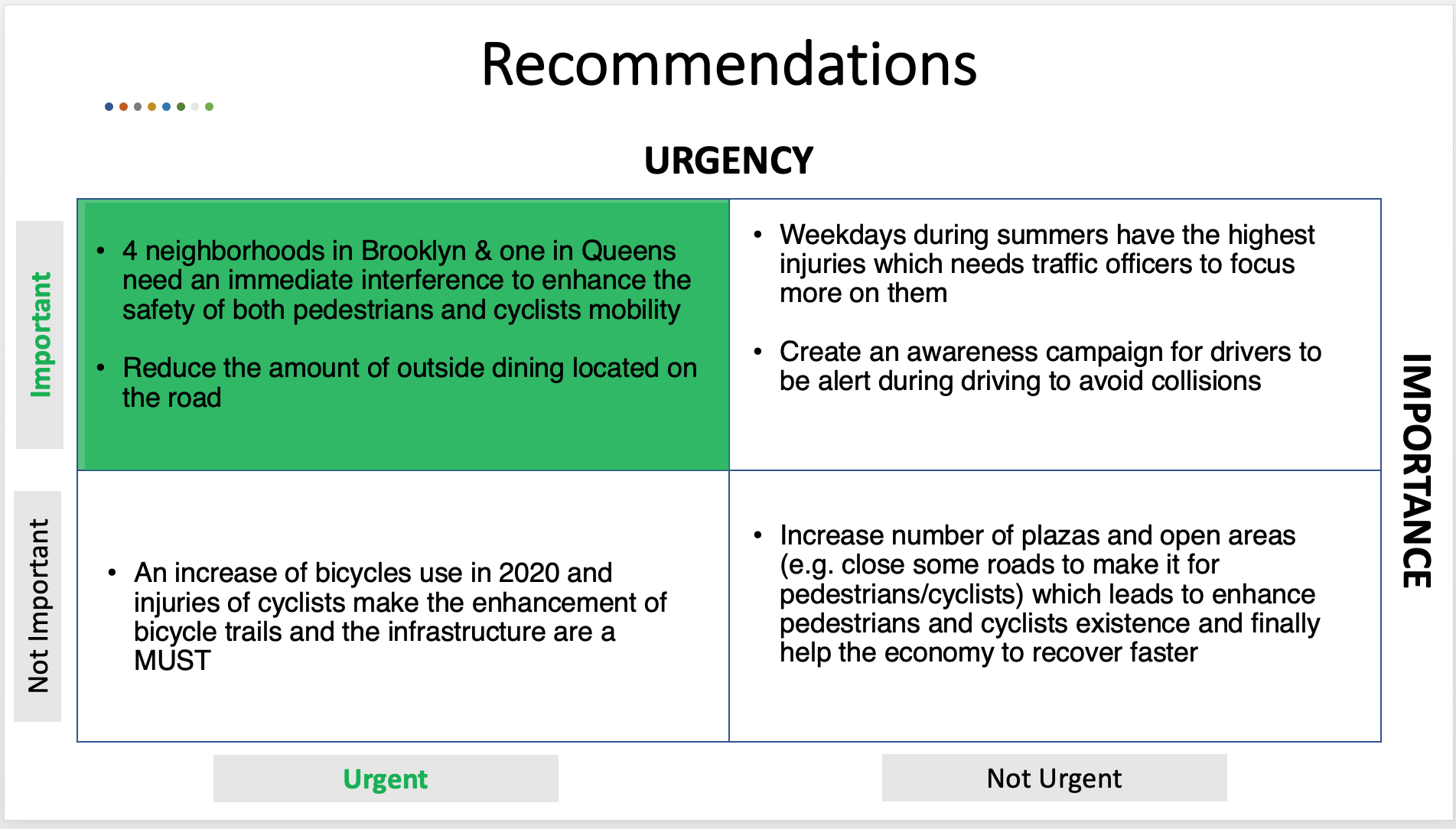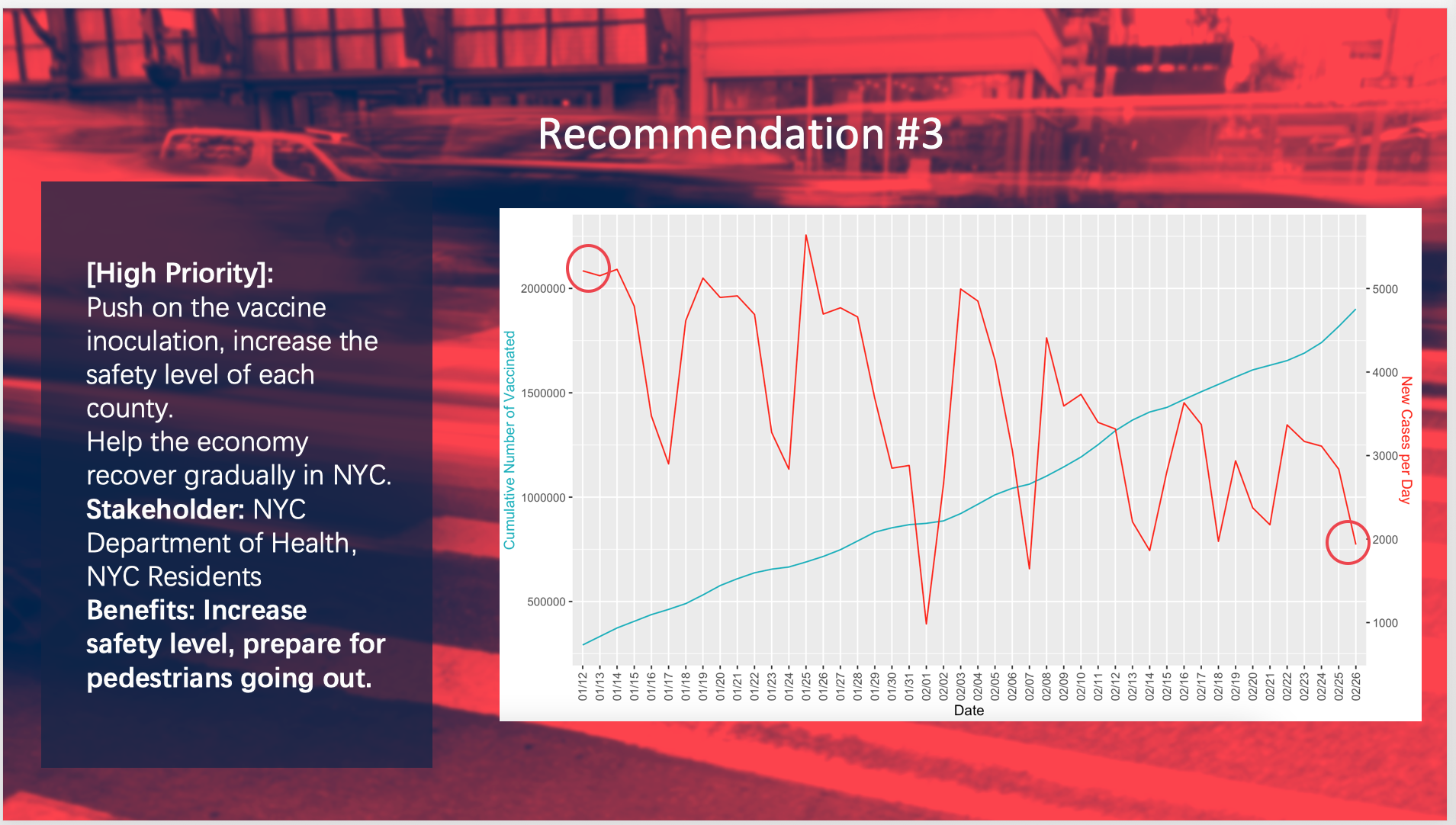Case 1: Professor Proposes (SOLUTION)
Share
Explore

#Solution and Approach

This case’s technical approach should take on over the following path:
Firstly, since students are using Excel, hence the number of “X” variables cannot be greater than 16, so a challenge is to reduce the number of “X” variables. Of course using other software packages one could use a lot of “X” variables (dummies for the categorical variables). However since Excel is being used so this is challenge number 1. The best way to handle is by transforming categorical variables into numerical. This way, you will remove number of new columns introduced by dummyfication
Using the context in the case, you can create a numerical table with scores for each of the class type in your categorical variables. For example: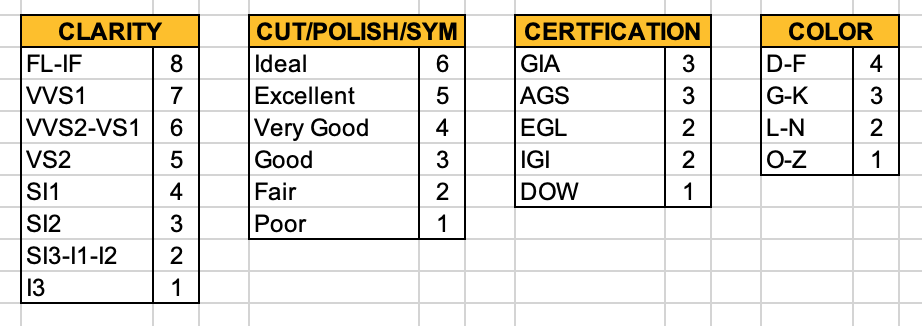Secondly, students have a tendency to straight away jump to “running” a regression model without properly taking a look at the data. In this case you would notice that there are three wholesalers that the Prof downloaded data from. Wholesaler sells only low carat/low price diamonds. This becomes evident if you do a scatter plot between “Price” and “Carat”. So the question then becomes should we be using all the data in a single regression model, in other words are there “structural” breaks in the data such that a single regression (linear regression) would be appropriate or not. This can be tested using a test of “model stability” such as the “Chow” test covered in a video lesson. One would notice that if you run a Chow test, the test does indicate that all three wholesalers cannot be included in a single regression model. Since the Prof’s diamond is of a higher carat weight that leads us to drop wholesaler 3 in developing a predictive model for the diamond price.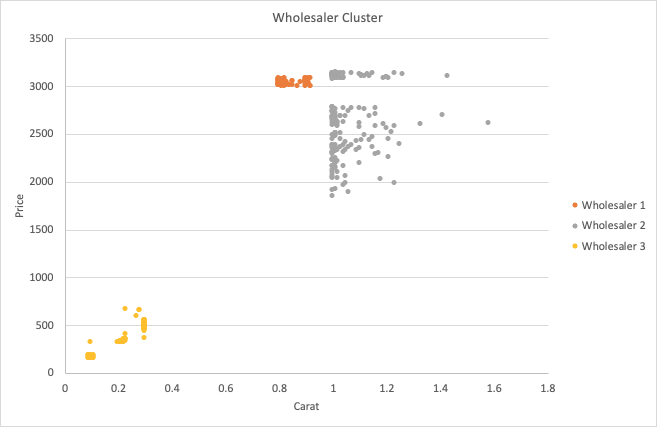Keep if mind that when you drop wholesaler 3 data from your model, the r-square substantially drops. But then this corresponds to the trade off between a “ better” fitting “incorrect” model versus a “less fitting” “correct” model for a prediction.
Using a “correct” model for a prediction with numerical variables for all categories, we yield the following results. This is not the only correct regression model. Models will variate depending on the handling of categorical variables. However, what we are looking for a prediction “the price is fair” as per the quote the professor has received.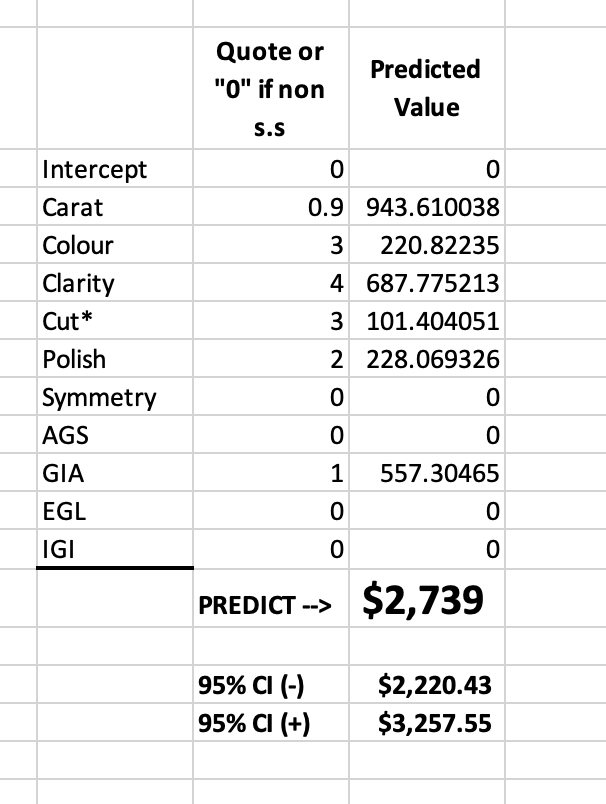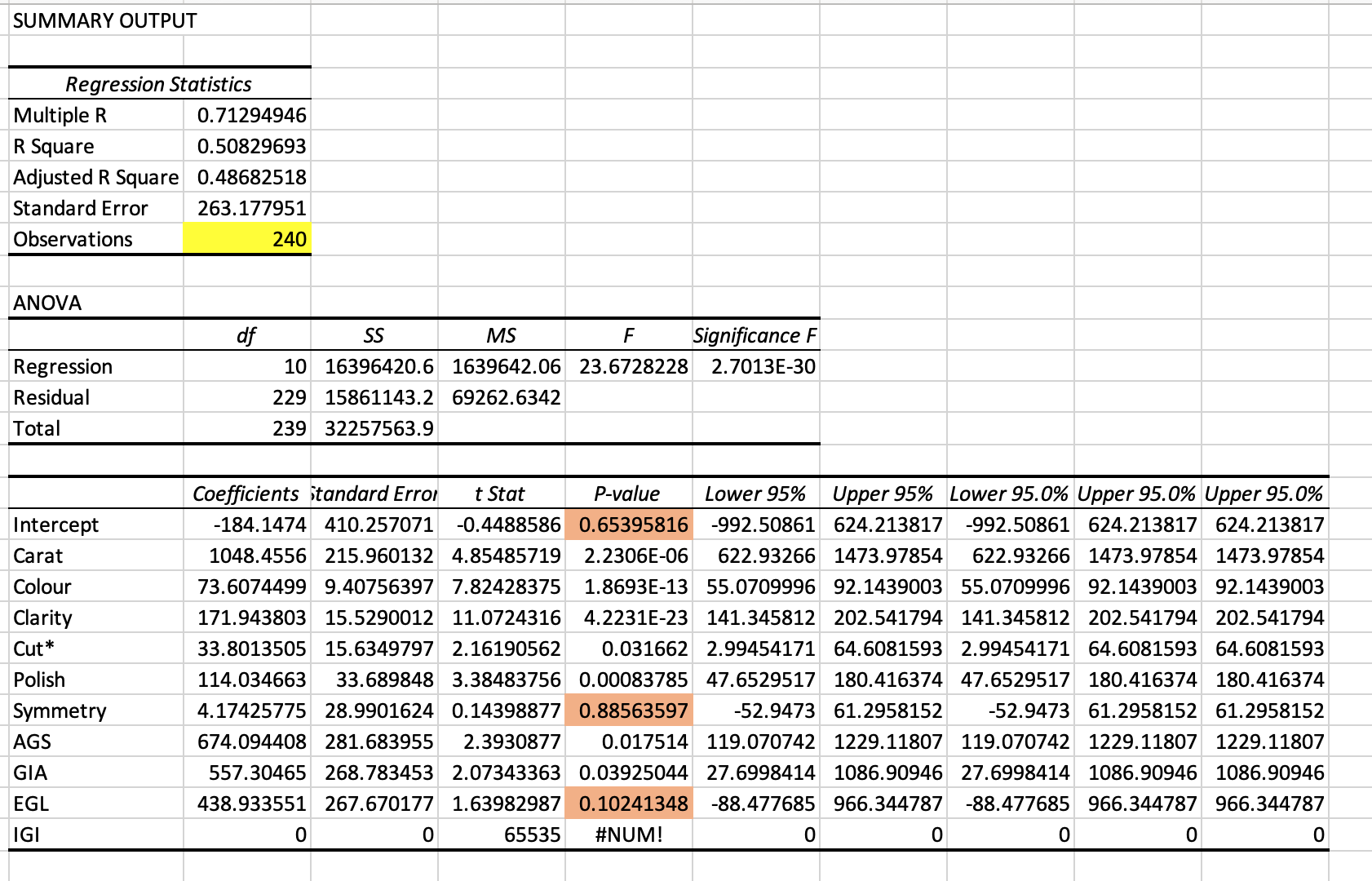Visual recommendations for EDA
Below are some slides of my current work where EDA and data-driven recommendation are produced.